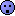# Thread: algebra inequality help

1. ## algebra inequality help

Hi, could you please help me solve this question? Thank you!If x and y are positive, which of the following must be greater than 1/sqrt(x+y) ?

1) (sqrt(x+y))/(2x)

2) (sqrt(x)+sqrt(y))/(x+y)

2) (sqrt(x)-sqrt(y))/(x+y)

2. 1) $\displaystyle \displaystyle\frac{\sqrt{x+y}}{2x}>\frac{1}{\sqrt{ x+y}}\Leftrightarrow x+y>2x\Leftrightarrow y>x$
So, the inequality is true iff $\displaystyle y>x$ and not for all positive x and y.

2) $\displaystyle \displaystyle\frac{\sqrt{x}+\sqrt{y}}{x+y}>\frac{1 }{\sqrt{x+y}}\Leftrightarrow\frac{\sqrt{x}+\sqrt{y }}{x+y}>\frac{\sqrt{x+y}}{x+y}\Leftrightarrow\sqrt {x}+\sqrt{y}>\sqrt{x+y}\Leftrightarrow 2\sqrt{xy}>0$
which is true for all positive x, y.

3) $\displaystyle \displaystyle\frac{\sqrt{x}-\sqrt{y}}{x+y}>\frac{1}{\sqrt{x+y}}$
Is not true if x<y, because LHM is negative and RHM is positive.

#### Search Tags

algebra, inequality